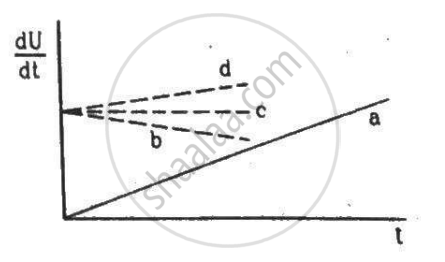Department of Pre-University Education, KarnatakaPUC Karnataka Science Class 12
Advertisement Remove all ads

# A Constant Current I Is Passed Through a Resistor. Taking the Temperature Coefficient of Resistance into Account - Physics

Short Note

A constant current i is passed through a resistor. Taking the temperature coefficient of resistance into account, indicate which of the plots shown in the figure best represents the rate of production of thermal energy in the resistor.Advertisement Remove all ads

#### Solution

Plot (d) is correct.

When current passes through a resistor, the temperature of the resistor increases due to the heat produced in it.
H = i2Rt,

where i = current flowing through the resistor

R = resistance of the resistor

t = time for which the current is flowing

With the increase in the temperature of the resistor, its resistance is also increased. The rate of production of thermal energy in the resistor of the circuit is given by the following relation:-

$\frac{dU}{dt} = \frac{d}{dt}( i^2 Rt) = i^2 R$

where i = current flowing through the resistor

R = resistance of the resistor

Rate of production of thermal energy in the resistor is directly proportional to the resistance. So, due to increase in resistance, $\frac{dU}{dt}$ also increases linearly, which is best represented by plot d.

Is there an error in this question or solution?
Advertisement Remove all ads

#### APPEARS IN

HC Verma Class 11, Class 12 Concepts of Physics Vol. 2
Chapter 11 Thermal and Chemical Effects of Current
MCQ | Q 2 | Page 217
Advertisement Remove all ads

#### Video TutorialsVIEW ALL 

Advertisement Remove all ads
Share
Notifications

View all notifications

Forgot password?